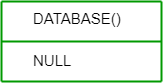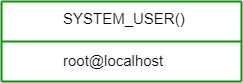GFG App
Open AppBrowser
Continue

SQL (Structured Query Language) offers a wide range of advanced functions that allow you to perform complex calculations, transformations, and aggregations on your data.

## Aggregate Functions

In database management an aggregate function is a function where the values of multiple rows are grouped together as input on certain criteria to form a single value of more significant meaning.

• SUM(): Calculates the sum of values in a column.
• AVG(): Computes the average of values in a column.
• COUNT(): Returns the number of rows or non-null values in a column.
• MIN(): Finds the minimum value in a column.
• MAX(): Retrieves the maximum value in a column.

## Conditional Functions

• CASE WHEN: Allows conditional logic to be applied in the SELECT statement.
• COALESCE(): Returns the first non-null value in a list.
• NULLIF(): Compares two expressions and returns null if they are equal; otherwise, returns the first expression.

## Mathematical Functions

Mathematical functions are present in SQL which can be used to perform mathematical calculations. Some commonly used mathematical functions are given below:

• ABS(): Returns the absolute value of a number.
• ROUND(): Rounds a number to a specified number of decimal places.
• POWER(): Raises a number to a specified power.
• SQRT(): Calculates the square root of a number.

BIN(): It converts a decimal number to a binary number.

Query:

`SELECT BIN(18);`

Output:BINARY(): It converts a value to a binary string.

Query:

`SELECT BINARY "GeeksforGeeks";`

Output:COALESCE(): It returns the first non-null expression in a list.

Query:

`SELECT COALESCE(NULL,NULL,'GeeksforGeeks',NULL,'Geeks');`

Output:CONNECTION_ID(): It returns the unique connection ID for the current connection.

Query:

`SELECT CONNECTION_ID();`

Output:CURRENT_USER(): It returns the user name and hostname for the MySQL account used by the server to authenticate the current client.

Query:

`SELECT CURRENT_USER();`

Output:DATABASE(): It returns the name of the default database.

Query:

`SELECT DATABASE();`

Output:IF(): It returns one value if a condition is TRUE, or another value if a condition is FALSE.

Query:

`SELECT IF(200<500, "YES", "NO");`

Output:LAST_INSERT_ID(): It returns the first AUTO_INCREMENT value that was set by the most recent INSERT or UPDATE statement.

Query:

`SELECT LAST_INSERT_ID();`

Output:Query:

`SELECT NULLIF(25.11, 25);`

Output:Query:

`SELECT NULLIF(115, 115);`

Output:SESSION_USER(): It returns the user name and host name for the current MySQL user.

Query:

`SELECT SESSION_USER();`

Output:SYSTEM_USER(): It returns the user name and host name for the current MySQL user.

Query:

`SELECT SYSTEM_USER();`

Output:USER(): It returns the user name and host name for the current MySQL user.

Query:

`SELECT USER();`

Output:VERSION(): It returns the version of the MySQL database.

Query:

`SELECT VERSION();`

Output:My Personal Notes arrow_drop_up